@Team 2018-09-02T14:45:08.000000Z 字数 15723 阅读 3495

# 卷积神经网络[AlexNet，VGG，GoodLeNet，resNet(还没写)，Xception，SqueezeNet,shufflenet(没写),MobileNet]

石文华

AlexNet，是以论文的第一作者 Alex Krizhevsky 的名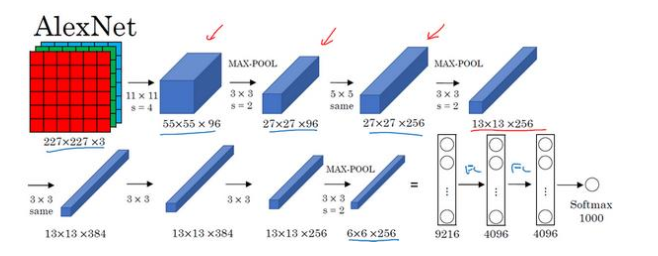（1）输入层：AlexNet 首先用一张 227×227×3 的图片作为输入，实际上原文中使用的图像是 224×224×3，但是如果你尝试去推导一下，你会发现 227×227 这个尺寸更好一些,这里使用输入数据是224*224*3的图像进行推导，即长宽都是224的图像，色彩通道是R、G、B三个。
（3）MaxPool1层：卷积之后使用最大池化，池化的滑动窗口是3*3*96，步长为2，池化之后输出的特征图的长宽是27,27=（55-3）/2+1，所以池化之后的特征图维度是27*27*96。
（5）MaxPool2层：滑动窗口大小为3*3*256，步长为2，得到的长宽为13,13=（27-3）/2+1,最后得到的特征图大小为13*13*256。
（9）MaxPool3:滑动窗口大小为3*3*256，步长为2，得到的长宽为6,6=（13-3）/2+1,最后得到的特征图大小为6*6*256。
（10）FC6层:第一个全连接层，输入特征维度是6*6*256，首先先对输入的特征图进行扁平化处理，将维度变为1*9216的输入特征图，因为要求输出的数据维度为1*4096，所以需要一个9216*4096的矩阵完成输入数据和输出数据的全连接，最后得到输出数据的维度为1*4096。
（11）FC7层：输入数据维度是1*4096，输出特征要求为1*4096，所以需要4096*4096的矩阵完成输入数据和输出数据的全连接，随后得到输出数据的维度是1*4096。
（12）FC8层：输入数据维度是1*4096，输出特征要求为1*1000，所以需要4096*1000的矩阵完成输入数据和输出数据的全连接，随后得到输出数据的维度是1*1000。
（13）OutPut层：输出图像对应的1000个类别的可能性值，将FC7层的输出数据传递到Softma激活函数中，能够得到1000个全新的输出值，这1000个输出值就是模型预测的输入图像对应1000个类别的可能性。
AlexNet 网络结构看起来相对复杂，包含约 6000 万个参数， 这些数字（55×55×96、 27×27×96、
27×27×256……） 都是 Alex Krizhevsky 及其合著者不得不给出的,。

import torchimport torch.nn as nnclass AlexNet(nn.Module):    def __init__(self, num_classes=10):        super(AlexNet, self).__init__()        self.features = nn.Sequential(            nn.Conv2d(3, 96, kernel_size=11, stride=4, padding=0),            nn.ReLU(inplace=True),            nn.MaxPool2d(kernel_size=3, stride=2),            nn.Conv2d(96, 256, kernel_size=5, padding=2, groups=2),            nn.ReLU(inplace=True),            nn.MaxPool2d(kernel_size=3, stride=2),            nn.Conv2d(256, 384, kernel_size=3, padding=1),            nn.ReLU(inplace=True),            nn.Conv2d(384, 384, kernel_size=3, padding=1, groups=2),            nn.ReLU(inplace=True),            nn.Conv2d(384, 256, kernel_size=3, padding=1, groups=2),            nn.ReLU(inplace=True),            nn.MaxPool2d(kernel_size=3, stride=2),        )        self.classifier = nn.Sequential(            nn.Linear(256 * 6 * 6, 4096),            nn.ReLU(inplace=True),            nn.Dropout(),            nn.Linear(4096, 4096),            nn.ReLU(inplace=True),            nn.Dropout(),            nn.Linear(4096, num_classes),        )    def forward(self, x):        x = self.features(x)        x = x.view(x.size(0), 256 * 6 * 6)        x = self.classifier(x)        return xalexnet=AlexNet()print(alexnet)

AlexNet(  (features): Sequential(    (0): Conv2d(3, 96, kernel_size=(11, 11), stride=(4, 4))    (1): ReLU(inplace)    (2): MaxPool2d(kernel_size=3, stride=2, padding=0, dilation=1, ceil_mode=False)    (3): Conv2d(96, 256, kernel_size=(5, 5), stride=(1, 1), padding=(2, 2), groups=2)    (4): ReLU(inplace)    (5): MaxPool2d(kernel_size=3, stride=2, padding=0, dilation=1, ceil_mode=False)    (6): Conv2d(256, 384, kernel_size=(3, 3), stride=(1, 1), padding=(1, 1))    (7): ReLU(inplace)    (8): Conv2d(384, 384, kernel_size=(3, 3), stride=(1, 1), padding=(1, 1), groups=2)    (9): ReLU(inplace)    (10): Conv2d(384, 256, kernel_size=(3, 3), stride=(1, 1), padding=(1, 1), groups=2)    (11): ReLU(inplace)    (12): MaxPool2d(kernel_size=3, stride=2, padding=0, dilation=1, ceil_mode=False)  )  (classifier): Sequential(    (0): Linear(in_features=9216, out_features=4096, bias=True)    (1): ReLU(inplace)    (2): Dropout(p=0.5)    (3): Linear(in_features=4096, out_features=4096, bias=True)    (4): ReLU(inplace)    (5): Dropout(p=0.5)    (6): Linear(in_features=4096, out_features=10, bias=True)  ))

import torch from torchvision import datasets,transformsimport osimport matplotlib.pyplot as pltimport time#transform = transforms.Compose是把一系列图片操作组合起来，比如减去像素均值等。#DataLoader读入的数据类型是PIL.Image#这里对图片不做任何处理，仅仅是把PIL.Image转换为torch.FloatTensor，从而可以被pytorch计算transform = transforms.Compose(    [        transforms.Scale([224,224]),        transforms.ToTensor(),        #transforms.Normalize(mean=[0.5,0.5,0.5],std=[0.5,0.5,0.5])    ])#训练集train_set = datasets.CIFAR10(root='drive/pytorch/Alexnet/', train=True, transform=transform, target_transform=None, download=True)#测试集test_set=datasets.CIFAR10(root='drive/pytorch/Alexnet/',train=False,download=True,transform=transform)trainloader=torch.utils.data.DataLoader(train_set,batch_size=32,shuffle=True,num_workers=0)testloader=torch.utils.data.DataLoader(test_set,batch_size=32,shuffle=True,num_workers=0)classes=('plane','car','bird','cat','deer','dog','frog','horse','ship','truck')(data,label)=train_setprint(classes[label])

X_example,y_example=next(iter(trainloader))print(X_example.shape)img=X_example.permute(0, 2, 3, 1)print(img.shape)import torchvisionimg=torchvision.utils.make_grid(X_example)img=img.numpy().transpose([1,2,0])import matplotlib.pyplot as pltplt.imshow(img)plt.show()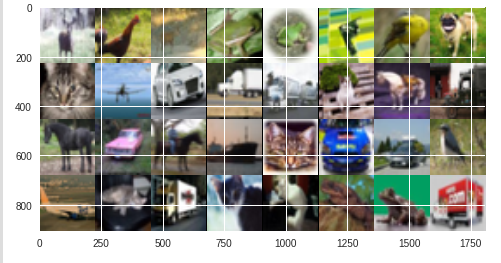import torch.optim as optim          #导入torch.potim模块import timefrom torch.autograd import Variable   # 这一步还没有显式用到variable，但是现在写在这里也没问题，后面会用到import torch.nn as nnimport torch.nn.functional as Fcriterion = nn.CrossEntropyLoss()    #同样是用到了神经网络工具箱 nn 中的交叉熵损失函数optimizer = optim.Adam(alexnet.classifier.parameters(), lr=0.0001)   #optim模块中的SGD梯度优化方式---随机梯度下降epoch_n=5for epoch in range(epoch_n):  print("Epoch{}/{}".format(epoch,epoch_n-1))  print("-"*10)  running_loss = 0.0  #定义一个变量方便我们对loss进行输出  running_corrects=0  for i, data in enumerate(trainloader, 1): # 这里我们遇到了第一步中出现的trailoader，代码传入    inputs, labels = data   # data是从enumerate返回的data，包含数据和标签信息，分别赋值给inputs和labels    #inputs=inputs.permute(0, 2, 3, 1)    #print("hahah",len(labels))    y_pred = alexnet(inputs)                # 把数据输进网络net，这个net()在第二步的代码最后一行我们已经定义了    _,pred=torch.max(y_pred.data,1)    optimizer.zero_grad()                # 要把梯度重新归零，因为反向传播过程中梯度会累加上一次循环的梯度    loss = criterion(y_pred, labels)    # 计算损失值,criterion我们在第三步里面定义了    loss.backward()                      # loss进行反向传播，下文详解    optimizer.step()                     # 当执行反向传播之后，把优化器的参数进行更新，以便进行下一轮    # print statistics                   # 这几行代码不是必须的，为了打印出loss方便我们看而已，不影响训练过程    running_loss += loss.item()       # 从下面一行代码可以看出它是每循环0-1999共两千次才打印一次    running_corrects+=torch.sum(pred==labels.data)    if(i % 2 == 0):    # print every 2000 mini-batches   所以每个2000次之类先用running_loss进行累加      print("Batch{},Train Loss:{:.4f},Train ACC:{:.4f}".format(i,running_loss/i,100*running_corrects/(32*i)))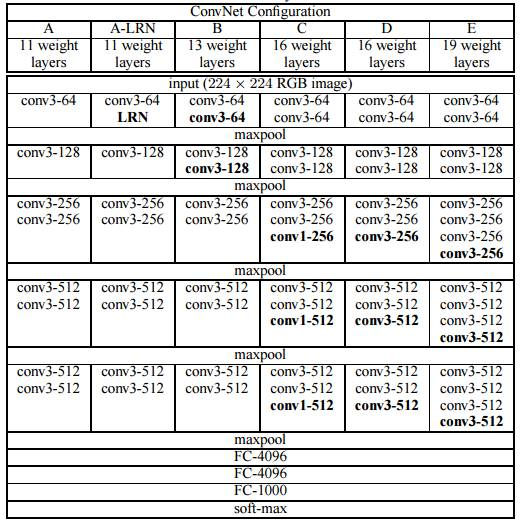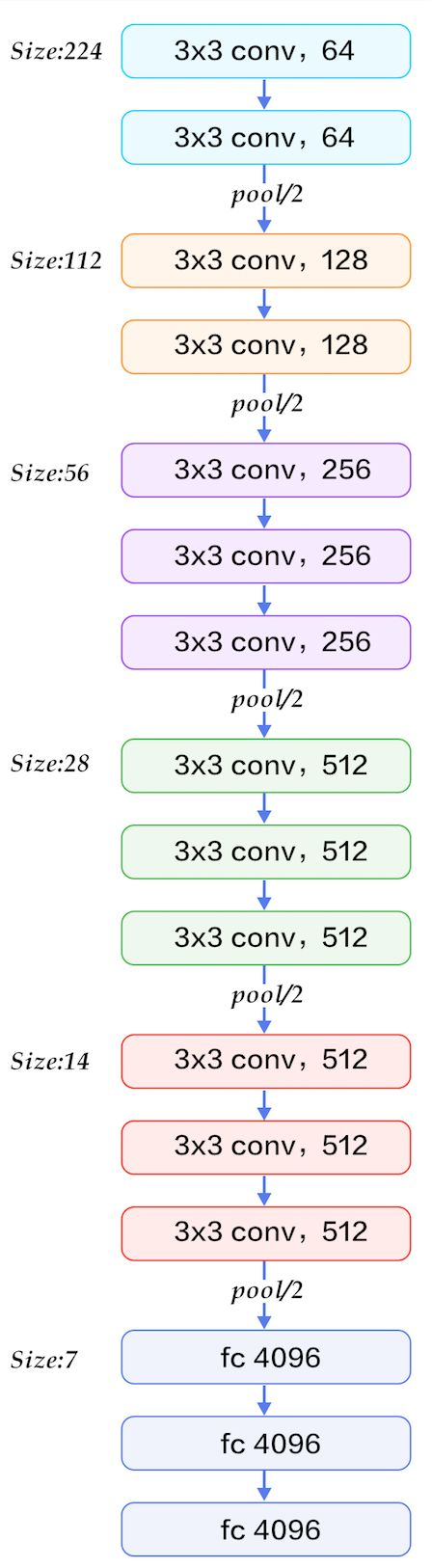VGG-16维度的变换情况可以参考AlexNet文中的方式进行推导。由图可以看到，VGG-16 是一种只需要专注于构建卷积层的简单网络。首

2×2，步幅为 2 的过滤器构建最大池化层。因此 VGG 网络的一大优点是它确实简化了神经网

import torchimport torch.nn as nnclass VGGNet16(nn.Module):    def __init__(self,num_classes=10):        super(VGGNet16,self).__init__()        self.Conv=nn.Sequential(            nn.Conv2d(3,64,kernel_size=3,stride=1,padding=1),            nn.ReLU(),            nn.Conv2d(64,64,kernel_size=3,stride=1,padding=1),            nn.ReLU(),            nn.MaxPool2d(kernel_size=2,stride=2),            nn.Conv2d(64,128,kernel_size=3,stride=1,padding=1),            nn.ReLU(),            nn.Conv2d(128,128,kernel_size=3,stride=1,padding=1),            nn.ReLU(),            nn.MaxPool2d(kernel_size=2,stride=2),            nn.Conv2d(128,256,kernel_size=3,stride=1,padding=1),            nn.ReLU(),            nn.Conv2d(256,256,kernel_size=3,stride=1,padding=1),            nn.ReLU(),            nn.Conv2d(256,256,kernel_size=3,stride=1,padding=1),            nn.ReLU(),            nn.MaxPool2d(kernel_size=2,stride=2),            nn.Conv2d(256,512,kernel_size=3,stride=1,padding=1),            nn.ReLU(),            nn.Conv2d(512,512,kernel_size=3,stride=1,padding=1),            nn.ReLU(),            nn.Conv2d(512,512,kernel_size=3,stride=1,padding=1),            nn.ReLU(),            nn.MaxPool2d(kernel_size=2,stride=2)        )        self.classifier = nn.Sequential(            nn.Linear( 6 * 6*512, 1024),            nn.ReLU(),            nn.Dropout(p=0.5),            nn.Linear(1024,1024),            nn.ReLU(),            nn.Dropout(p=0.5),            nn.Linear(1024, num_classes),         )        def forward(self,inputs):            x=self.Conv(inputs)            x=x.view(-1,4*4*512)            x=self.classifier(x)            return xvgg=VGGNet16()print(vgg)

VGGNet16(  (Conv): Sequential(    (0): Conv2d(3, 64, kernel_size=(3, 3), stride=(1, 1), padding=(1, 1))    (1): ReLU()    (2): Conv2d(64, 64, kernel_size=(3, 3), stride=(1, 1), padding=(1, 1))    (3): ReLU()    (4): MaxPool2d(kernel_size=2, stride=2, padding=0, dilation=1, ceil_mode=False)    (5): Conv2d(64, 128, kernel_size=(3, 3), stride=(1, 1), padding=(1, 1))    (6): ReLU()    (7): Conv2d(128, 128, kernel_size=(3, 3), stride=(1, 1), padding=(1, 1))    (8): ReLU()    (9): MaxPool2d(kernel_size=2, stride=2, padding=0, dilation=1, ceil_mode=False)    (10): Conv2d(128, 256, kernel_size=(3, 3), stride=(1, 1), padding=(1, 1))    (11): ReLU()    (12): Conv2d(256, 256, kernel_size=(3, 3), stride=(1, 1), padding=(1, 1))    (13): ReLU()    (14): Conv2d(256, 256, kernel_size=(3, 3), stride=(1, 1), padding=(1, 1))    (15): ReLU()    (16): MaxPool2d(kernel_size=2, stride=2, padding=0, dilation=1, ceil_mode=False)    (17): Conv2d(256, 512, kernel_size=(3, 3), stride=(1, 1), padding=(1, 1))    (18): ReLU()    (19): Conv2d(512, 512, kernel_size=(3, 3), stride=(1, 1), padding=(1, 1))    (20): ReLU()    (21): Conv2d(512, 512, kernel_size=(3, 3), stride=(1, 1), padding=(1, 1))    (22): ReLU()    (23): MaxPool2d(kernel_size=2, stride=2, padding=0, dilation=1, ceil_mode=False)  )  (classifier): Sequential(    (0): Linear(in_features=18432, out_features=1024, bias=True)    (1): ReLU()    (2): Dropout(p=0.5)    (3): Linear(in_features=1024, out_features=1024, bias=True)    (4): ReLU()    (5): Dropout(p=0.5)    (6): Linear(in_features=1024, out_features=1000, bias=True)  ))

GoodLeNet在2014年举办的ILSVRC中获得了分类任务第一名，与同年在分类任务中获得亚军的VGGNet模型相比，它的深度更深，达到22层。该网络引入Inception模块，Inception能够代替人工来确定卷积层中的过滤器类型，或者确定是否需要创建卷积层或者池化层，比如，在Alexnet和vggnet中，可以看到使用过11*11、5*5、3*3的卷积核，这些卷积核的大小都是我们人工选定的。
1、Inception单元结构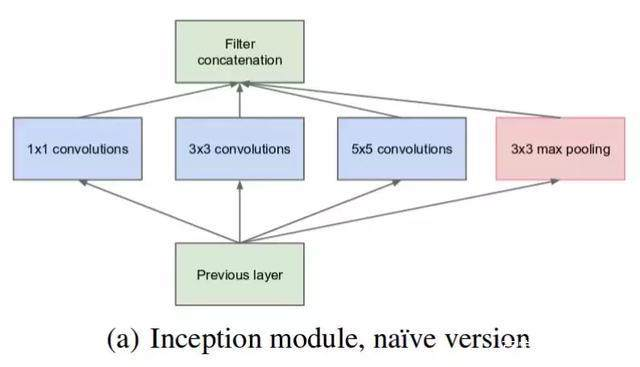（1）使用64个1*1卷积核，输出结果会是28*28*64；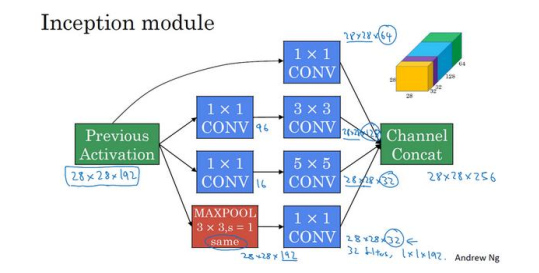（5×5×192）乘以输出值个数（28×28×32），把这些数相乘结果等于 1.2 亿（120422400） 。运算成本是非常高的。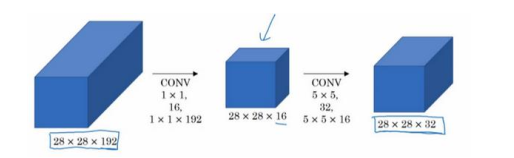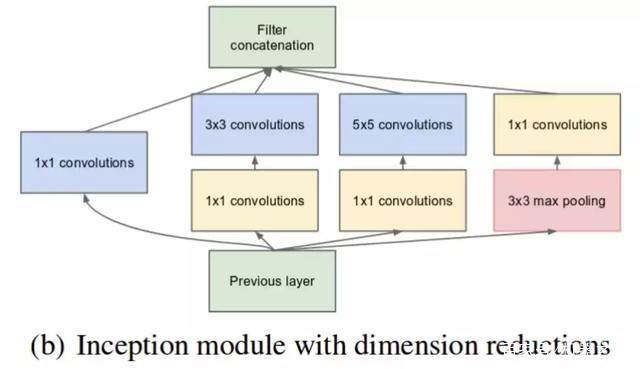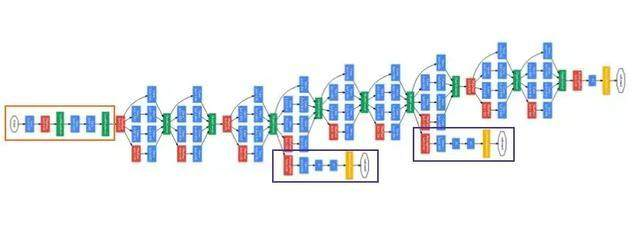2、Inception V2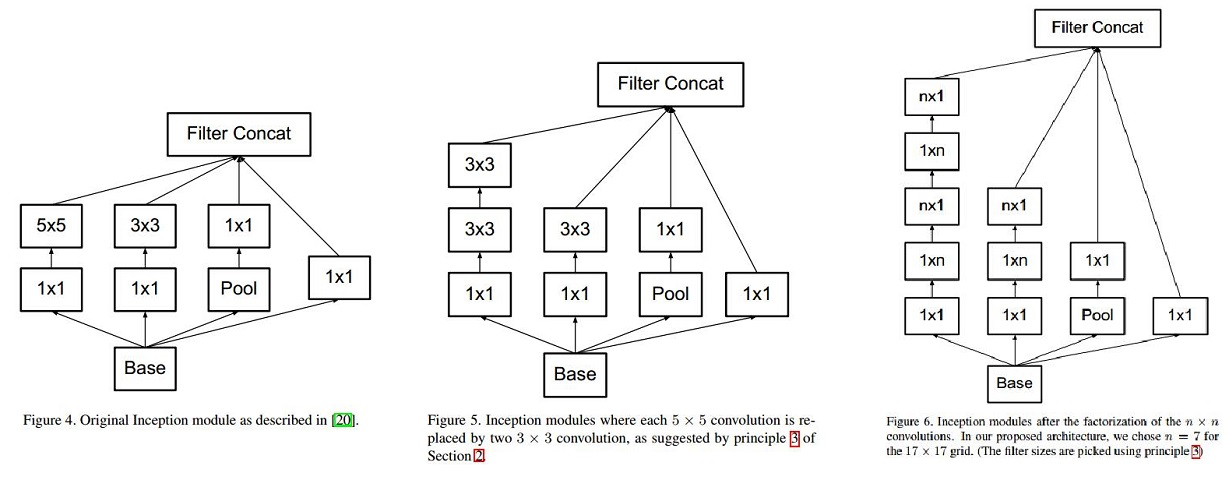3、Inception v3
V3整合了前面v2 中提到的所有升级，还使用了RMSProp 优化器、Factorized 7x7 卷积、辅助分类器使用了 BatchNorm；标签平滑（添加到损失公式的一种正则化项，旨在阻止网络对某一类别过分自信，即阻止过拟合）

from torchvision import dmodelsinceptionv3=models.inception_v3(pretrained=True)print(inceptionv3)

Xception目的不在于模型压缩，而是提高性能，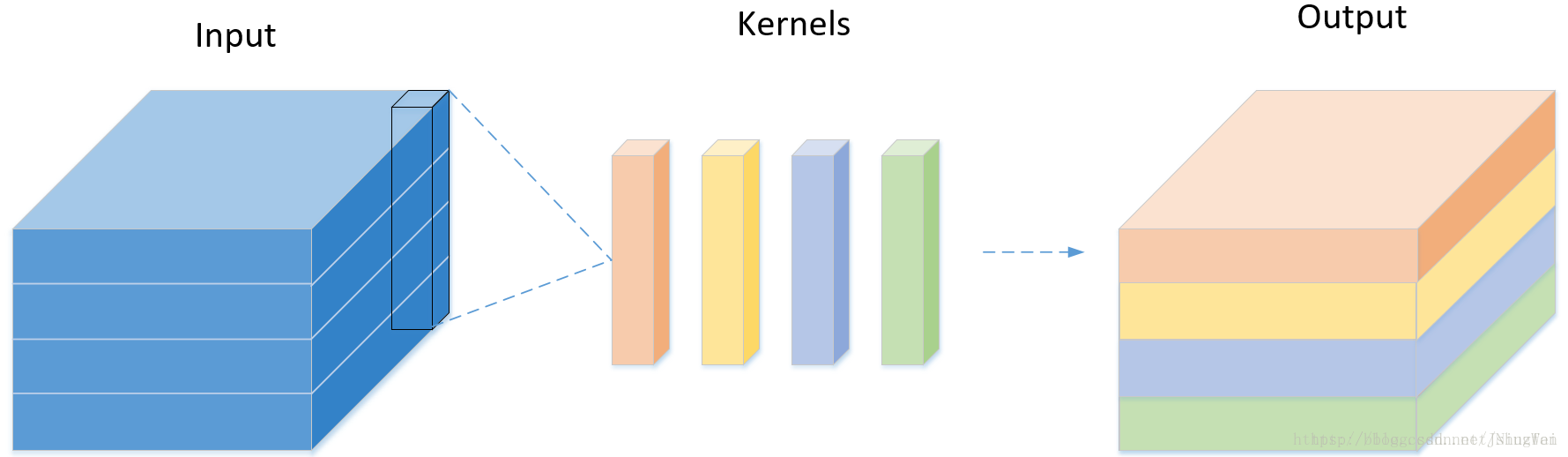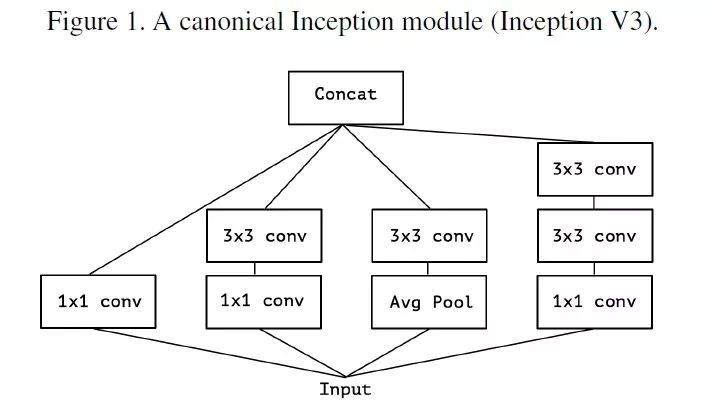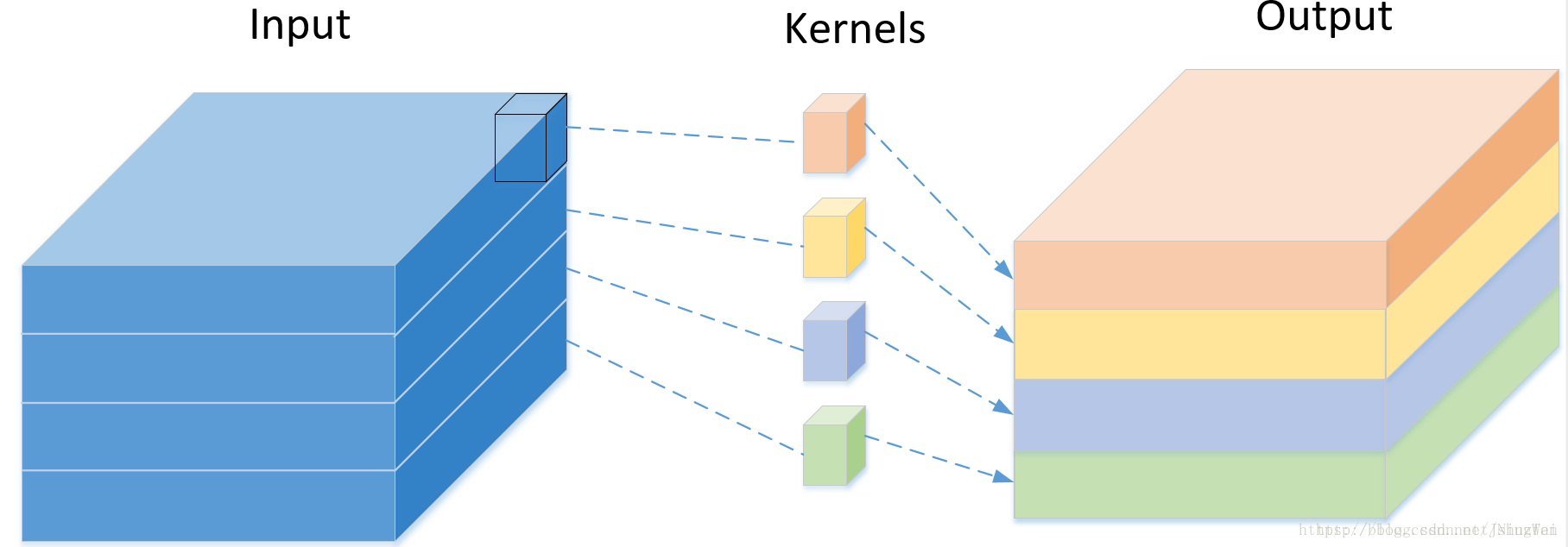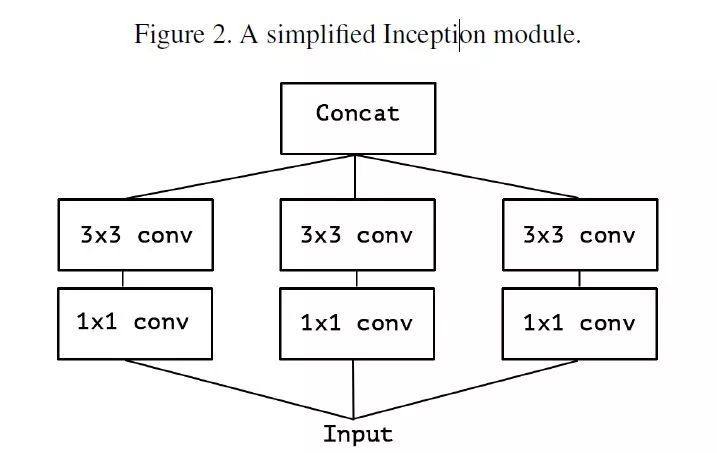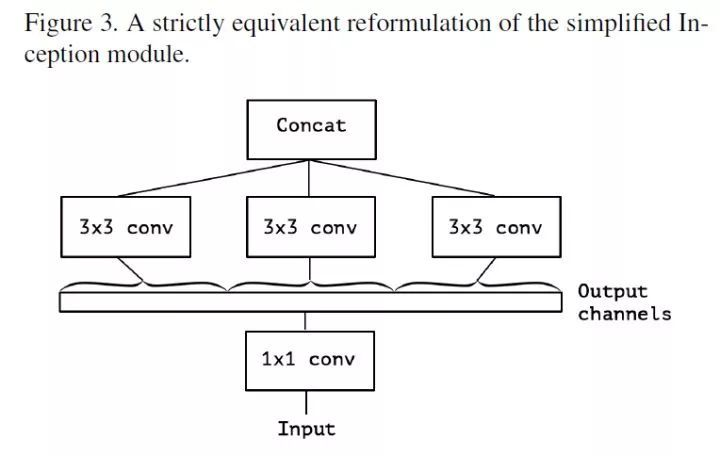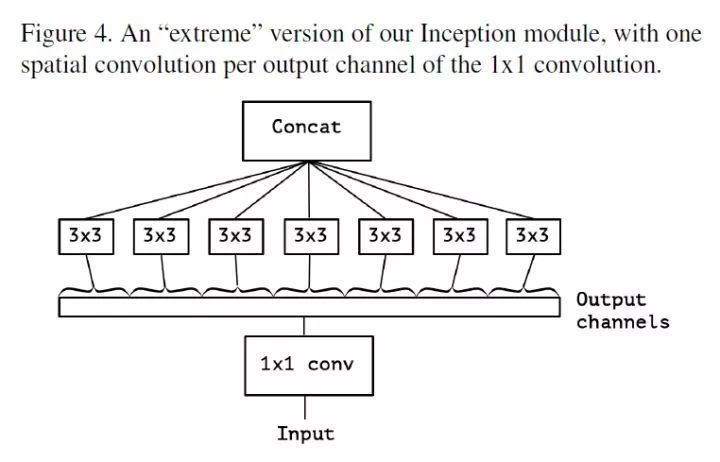keras.applications.xception.Xception(include_top=True, weights='imagenet',
input_tensor=None, input_shape=None,
pooling=None, classes=1000)

https://blog.csdn.net/jningwei/article/details/80628139
https://arxiv.org/pdf/1610.02357.pdf
https://www.sohu.com/a/226395640_500659
https://www.leiphone.com/news/201708/KGJYBHXPwsRYMhWw.html

1、概述：

2、设计此网络采用的策略：
（1）用1x1卷积核替换3x3卷积核，通道数相同的情况下，1x1的卷积核参数要比3x3的卷积核减少9倍。
（2）减少输入特征图的通道数，因为普通卷积的卷积核是：长通道，使用瓶颈层减少通道数的话参数就自然少了很多。
（3）延迟下采样，以使卷积层具有较大的激活的特征图。
3、网络的核心模块（Fire模块）
Fire有：首先是squeeze 卷积层组成（只有1x1过滤器），接着是由1x1和3x两种卷积都有的混合层（expand层）如下图所示：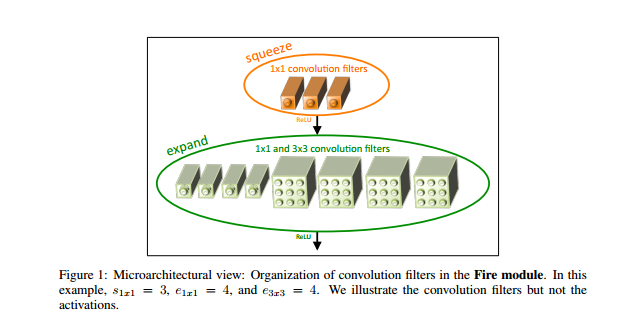4、网络结构

。根据策略3，需要延迟下采样，所以SqueezeNet分别在图层conv1，fire4，fire8和conv10以步幅2执行最大池化，尽可能使卷积层具有较大的特征图;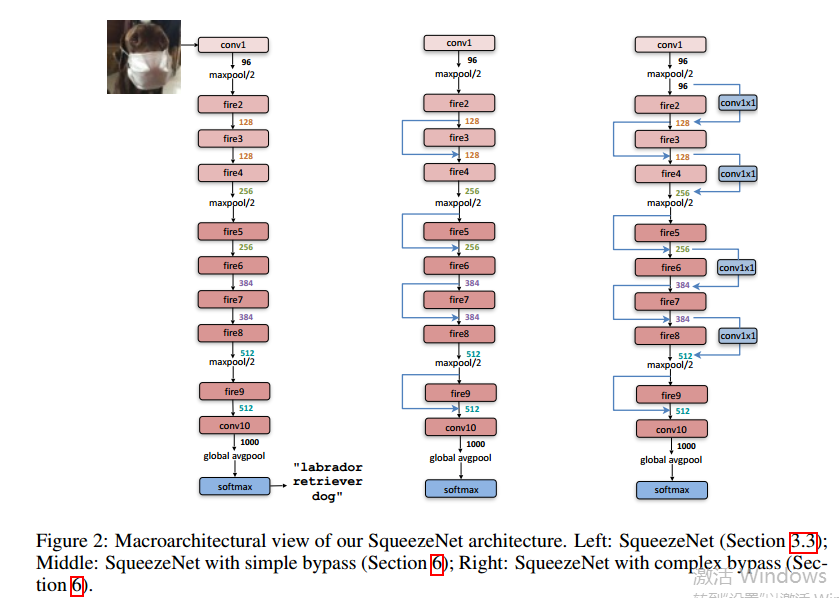（1）1x1和3x3卷积核的输出激活具有相同的高度和宽度，因此需要对3x3卷积前的特征图进行填充，使输出特征图跟输入特征图大小相同。
（2）Fire模块里面的挤压层和拓展层采用Relu函数进行激活
（3）在Fire9模块之后应用Dropout,比例为50%，
（4）没有全连接层
（5）在训练SqueezeNet时，学习率从0.04开始，并且在整个训练中线性降低学习率。

1、移动端和其他嵌入式系统通常是内存空间小，能耗要求低的，也就是计算资源有限。一般的模型，比如ImageNet数据集上训练的VGG,goodlenet,resnet等，需要巨大的计算资源，所以很难用在嵌入式系统上。
2、深度可分离卷积(depthwise separable convolution)
MobileNet使用技术之一是深度可分离卷积(depthwise separable convolution)代替传统的3D卷积操作，这样可以减少参数数量以及卷积过程中的计算量，但是也会导致特征丢失，使得精度下降。MobileNets其实就是Exception思想的应用。区别就是Exception文章重点在提高精度，而MobileNets重点在压缩模型。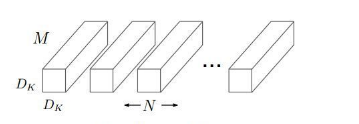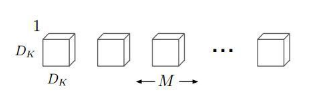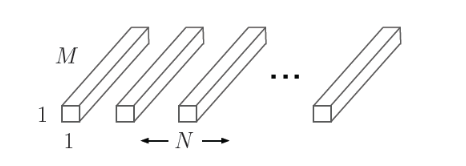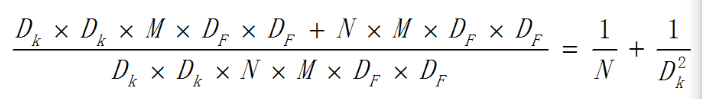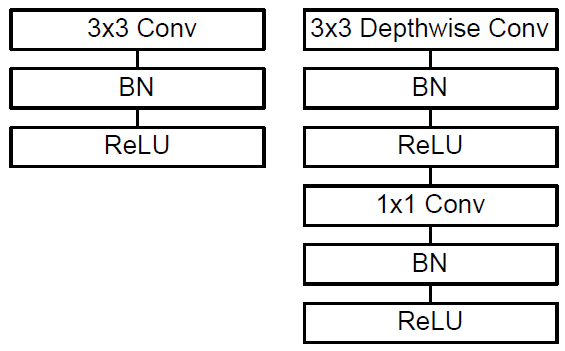3、两个超参数：宽度因子和分辨率因子
（1）宽度因子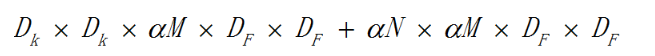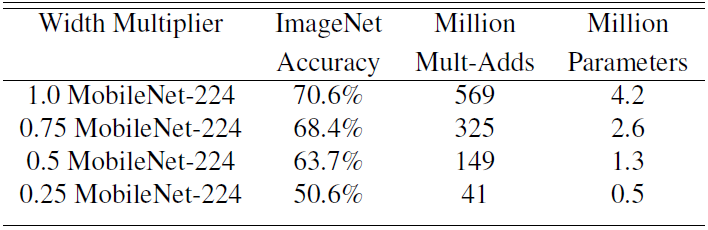（2）分辨率因子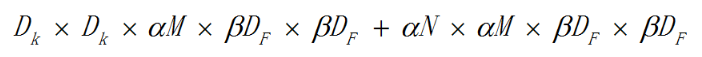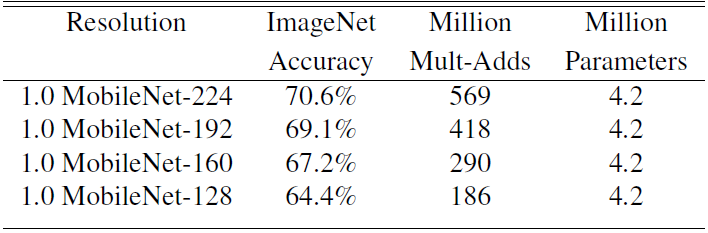4、改进：MobileNet V2

V1与V2的结构对比：（1）改进一：
V2在 DW 卷积之前新加了一个 PW 卷积，主要目的是为了提升维度数。相比V1直接在每个通道上单独提取特征，V2的这种做法能够先组合不同深度上的特征，并升维，使得特征更加丰富。比直接DW的话，特征提取的效果更好。
（2）改进二：
V2 去掉了第二个 PW 的激活函数。论文作者称其为 Linear Bottleneck。这么做的原因，是因为作者认为激活函数在高维空间能够有效的增加非线性，而在低维空间时则会破坏特征，由Relu的性质，Relu对于负的输入，输出全为零，所以relu会使得一部分特征失效。由于第二个 PW 的主要功能就是降维，再经过Relu的话，又要“损失”一部分特征，因此按照上面的理论，降维之后就不宜再使用 Relu了。
（3）使用短路连接: 倒残差(Inverted Residual)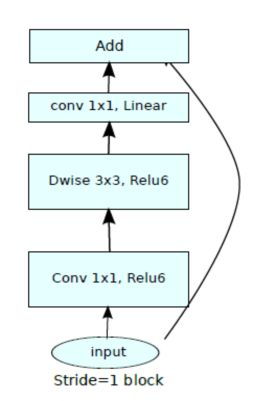https://blog.csdn.net/t800ghb/article/details/78879612
https://www.cnblogs.com/CodingML-1122/p/9043078.html
https://mp.weixin.qq.com/s/YhBvTLOliXzOw_7QEEtaKw
https://blog.csdn.net/u011995719/article/details/79135818
https://zhuanlan.zhihu.com/p/33075914
https://zhuanlan.zhihu.com/p/39386719• 私有
• 公开
• 删除